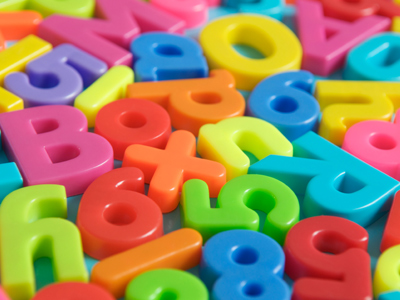Test your maths skills in this quiz.

# Level 3-4 Algebra - Putting Numbers in Place of Letters

By now you'll have realised that algebra plays an important part in KS3 Maths. In algebra, letters, such as a and b, are used in place of numbers. If the values of the letters are not known then they are called variables. But sometimes we do know what numbers the letters represent, or can work them out. This forms the heart of equations.

An equation is formed when an expression is given a value: that is, the expression equals something. Solving an equation involves finding out what value the letter has. That is a major part of maths so you'll need to be able to do it. But in this quiz things are simpler.

In the ten questions that follow you'll be given the value of certain letters. It's then up to you to use this information to answer the question. It's all good practice which will help you in the field of algebra.

1.
If a = 2, b = 3, c = 4 and d = 5, what is the value of 2a + 3b + c2 - d
24
26
28
30
4 + 9 + 16 - 5 = 24. Don't forget 'IF IN DOUBT, WRITE IT DOWN AND WORK IT OUT!
2.
If x = 5 and y = 10, what is the value of xy / 10
3
5
10
20
Two letters together need to be multiplied together
3.
If a = 2 and b = 3, what is the value of a + b + a?
5
6
7
8
2 + 3 + 2 = 7
4.
If a = 4 and b = 2, what is the value of a2 / b?
8
16
4
2
16 / 2 = 8
5.
If a = 6 and x = 8, which of the following gives a value of 22?
a + x2
a + 2x
2a + 2x
2ax
'If in doubt, write it down and work it out'
6.
If x = 2 and b = 4, what is the value of x2 + b2?
12
16
20
24
(2 x 2) + (4 x 4)
7.
If a = 4 and b = 5, what is the value of 2a + b
7
9
11
13
Remember that when a number and a letter are together they need to be multiplied together
8.
If x = 6 and y = 7, which of the following gives a value of 55
x2 + y2
xy2
x + y2
2x + 2y
You can make an estimate before you find the right answer. x2 + y2 would be too high, as would xy2. You can then check the other two more likely answers
9.
If x = 9 and y = 23, what is the value of y - x?
14
16
18
20
23 - 9 = 14
10.
If a = 4 and b = 8, what is the value of 2a + x2
64
75
80
It is impossible to say
Notice that we did not give you a value for x and therefore you cannot work out the expression!
You can find more about this topic by visiting BBC Bitesize - Writing and interpreting algebraic expressions

Author:  Frank Evans